##### Question

In: Statistics and Probability

# Question 1: Assume that the random variable X is normally​ distributed, with mean that = 47...

Question 1: Assume that the random variable X is normally​ distributed, with mean that = 47 and standard deviation that = 7. Compute the probability. Be sure to draw a normal curve with the area corresponding to the probability shaded.

P(X< AND = TO 43)

Using technology, what is P(X< AND = TO 43) equal? (round to four decimal places)

• How did you find this answer using a graphing calculator??

Question 2: The mean incubation time for a type of fertilized egg kept at 100.8​°F is 22 days. Suppose that the incubation times are approximately normally distributed with a standard deviation of 2 days.

(a) What is the probability that a randomly selected fertilized egg hatches in less than 20 ​days?

​(b) What is the probability that a randomly selected fertilized egg takes over 24 days to​ hatch?

​(c) What is the probability that a randomly selected fertilized egg hatches between 18 and 22 ​days?

​(d) Would it be unusual for an egg to hatch in less than 17 ​days? Why?

• How did you find these answers? Thanks!

## Solutions

##### Expert Solution

Since the random variable X is normally distributed, with mean that = 47 and standard deviation that = 7 hence

to find

a)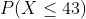is calculated using the Z score calculator as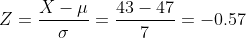hence

P(X</=43)=P(Z</=-0.57)

The probability value is calculated using the Z table shown below as

P-value=0.2843

b) since the mean incubation time for a type of fertilized egg kept at 100.8 °F is 22 days. Suppose that the incubation times are approximately normally distributed with a standard deviation of 2 days.

hence,

a) P(X<20)

Using Z formula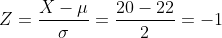P(Z<-1)=0.1587 using Z table

b) P(X.>24)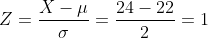P(Z>1)=0.1587 again computed using the Z table shown below

c) P(18<X<22)

Z at X=18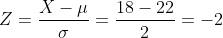and Z at X=22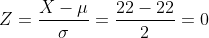So, P(10<X<22)

=P(-2<Z<0)

=0.50-0.0228

=0.4772

d) Again P(X<17)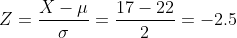According to Central limit theorem if Z value is less than -2 and greater than 2 then by rare event rule the probability of happening that event becomes very low hence it is unusual.

Z table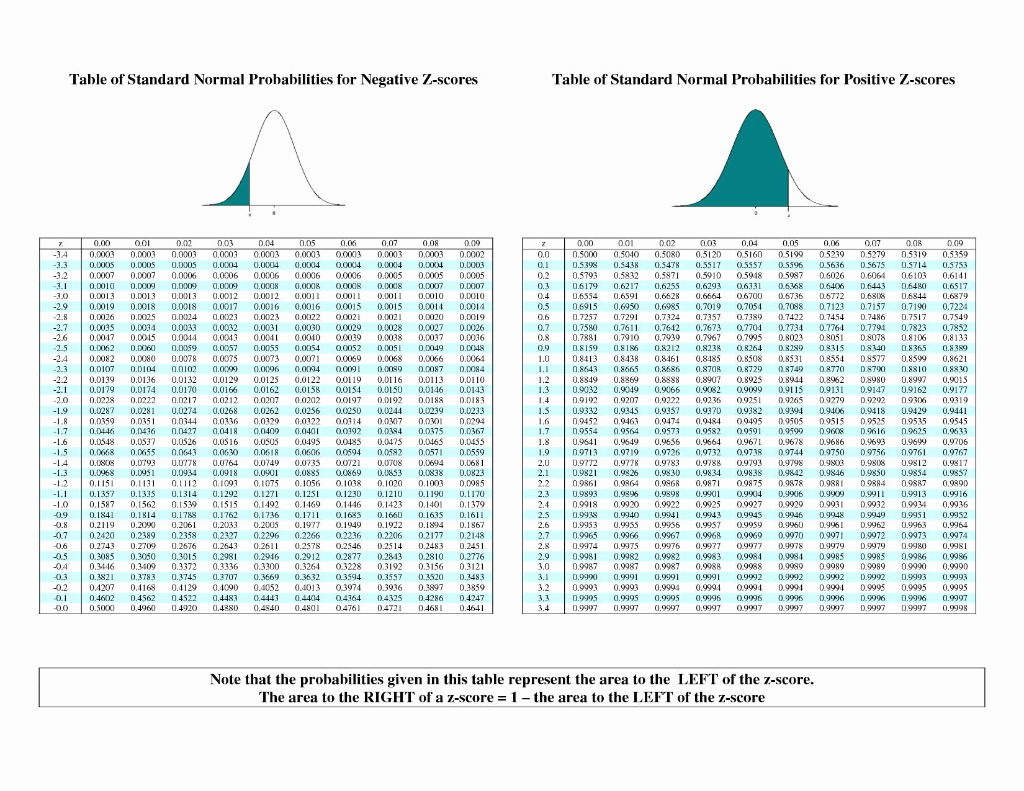## Related Solutions

##### Assume that the random variable x is normally distributed with mean μ = 70 and standard...
Assume that the random variable x is normally distributed with mean μ = 70 and standard deviation σ = 10. Find P(x < 82) a. 0.9942 b. 0.4500 c. 0.1151 d. 0.8849
##### Assume the random variable X is normally distributed with mean μ = 50 and standard deviation...
Assume the random variable X is normally distributed with mean μ = 50 and standard deviation σ = 7. Compute the probability. P(35<X<63)
##### Assume that the random variable X normally distributed, with mean 90 and standard deviation 15. Compute...
Assume that the random variable X normally distributed, with mean 90 and standard deviation 15. Compute the probability P(X>102).
##### Let x be a continuous random variable that is normally distributed with a mean of 65...
Let x be a continuous random variable that is normally distributed with a mean of 65 and a standard deviation of 15. Find the probability that x assumes a value: less than 48 greater than 87             between 56 and 70
##### 5. X is a normally distributed random variable with a mean of 8 and a standard...
5. X is a normally distributed random variable with a mean of 8 and a standard deviation of 3. The probability that X is between 6 and 10 is a. 0.7486 b. 0.4972 c. 0.6826 d. 0.8413 The weight of football players is normally distributed with a mean of 200 pounds and a standard deviation of 20 pounds. 6. The probability of a player weighing more than 240 pounds is a. 0.0197 b. 0.9803 c. 0.4803 d. 0.0228 7. Refer...
##### Suppose that y = x2, where x is a normally distributed random variable with a mean
Suppose that y = x2, where x is a normally distributed random variable with a mean and variance of µx = 0 and σ2x = 4. Find the mean and variance of y by simulation. Does µy = µ2x? Does σy = σ2x? Do this for 100, 1000, and 5000 trials.
##### A random variable X is Normally distributed with mean = 75 and = 8. Let Y...
A random variable X is Normally distributed with mean = 75 and = 8. Let Y be a second Normally distributed random variable with mean = 70 and = 12. It is also known that X and Y are independent of one another. Let W be a random variable that is the difference between X and Y (i.e., W = X – Y). What can be said about the distribution of W?
##### 1)If a variable X is normally distributed with a mean of 60 and a standard deviation...
1)If a variable X is normally distributed with a mean of 60 and a standard deviation of 15, what is P(X > 60) P(X > 75) P(X > 80) P(X < 50) P(45 < X < 75) P(X < 45)
##### Suppose a random variable X is normally distributed with mean 65.9 and standard deviation 9.5. Answer...
Suppose a random variable X is normally distributed with mean 65.9 and standard deviation 9.5. Answer the following questions: P(42.15 < X < 77.30) = [round to 4 decimal places] Tries 0/5 P(X ≤ 73.50) = [round to 4 decimal places] Tries 0/5 P(X = 77.30) = [round to 4 decimal places] Tries 0/5 Suppose a is such that: P(X ≤ a) = 0.49. Then a = [round to 2 decimal places] Tries 0/5 What is the IQR (inter-quartle range)...
##### Suppose a random variable X is normally distributed with mean 65.3 and standard deviation 9.4. Answer...
Suppose a random variable X is normally distributed with mean 65.3 and standard deviation 9.4. Answer the following questions: 1. P(47.44 < X < 76.58) = [round to 4 decimal places] 2. P(X ≤ 46.50) = [round to 4 decimal places] 3. P(X = 76.58) = [round to 4 decimal places] 4. Suppose a is such that: P(X ≤ a) = 0.39. Then a = [round to 2 decimal places] 5. What is the IQR (inter-quartle range) of X? [round...